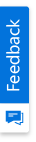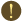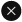We use cookies to give you the best experience on our website. If you continue to browse, then you agree to our privacy policy and cookie policy.Unfortunately, activation email could not send to your email. Please try again.# How to get the HubTile position when mouse clicked in WinForms HubTile control?

Platform: WinForms |
Control: HubTile

## HubTile position

The HubTile control contains Win 8 live tiles like, sliding functionality upon mouse selection on it. And now, there is no direct option to retrieve the sliding direction. That is, whether the sliding direction is left, right, top, bottom or middle in HubTile.

You can retrieve the sliding direction by handling its MouseDown event. The following code example demonstrates the same.

C#

```//HubTile rectangle
HubtileRect = new Rectangle(0, 0, this.hubTile1.Width, this.hubTile1.Height);
void hubTile1_MouseDown(object sender, MouseEventArgs e)
{
//Calculating Left side HubTile bounds
Rectangle LeftRectangle = new Rectangle(HubtileRect.X, HubtileRect.Y,     this.HubtileRect.Width / 4, this.HubtileRect.Height);
//Calculating Top side HubTile bounds
Rectangle UpRectangle = new Rectangle(LeftRectangle.Right, this.HubtileRect.Y, (this.HubtileRect.Width - (2 * (this.HubtileRect.Width / 4))), this.HubtileRect.Height / 4);
//Calculating Right side HubTile bounds
Rectangle RightRectangle = new Rectangle(UpRectangle.Right, this.HubtileRect.Y, this.HubtileRect.Width / 4, this.HubtileRect.Height);
//Calculating Bottom side HubTile bounds
Rectangle DownRectangle = new Rectangle(LeftRectangle.Right, (this.HubtileRect.Height / 2 + this.HubtileRect.Height / 4), (this.HubtileRect.Width - (2 * (this.HubtileRect.Width / 4))), HubtileRect.Height / 4);
if (LeftRectangle.Contains(e.Location))
{
Left = Right = Middle = Top = Bottom = false;
Left = true;
this.label1.Text = "Left";
}
else if (UpRectangle.Contains(e.Location))
{
Left = Right = Middle = Top = Bottom = false;
Top = true;
this.label1.Text = "Up";
}
else if (RightRectangle.Contains(e.Location))
{
Left = Right = Middle = Top = Bottom = false;
Right = true;
this.label1.Text = "Right";
}
else if (DownRectangle.Contains(e.Location))
{
Left = Right = Middle = Top = Bottom = false;
Bottom = true;
this.label1.Text = "Down";
}
else
{
Left = Right = Middle = Top = Bottom = false;
Middle = true;
this.label1.Text = "Middle";
}
}
```

VB

```'HubTile rectangle
HubtileRect = New Rectangle(0, 0, Me.hubTile1.Width, Me.hubTile1.Height)
Private Sub hubTile1_MouseDown(ByVal sender As Object, ByVal e As MouseEventArgs)
'Calculating Left side HubTile bounds
Dim LeftRectangle As New Rectangle(HubtileRect.X, HubtileRect.Y,  Me.HubtileRect.Width \ 4, Me.HubtileRect.Height)
'Calculating Top side HubTile bounds
Dim UpRectangle As New Rectangle(LeftRectangle.Right, Me.HubtileRect.Y, (Me.HubtileRect.Width - (2 * (Me.HubtileRect.Width \ 4))), Me.HubtileRect.Height \ 4)
'Calculating Right side HubTile bounds
Dim RightRectangle As New Rectangle(UpRectangle.Right, Me.HubtileRect.Y, Me.HubtileRect.Width \ 4, Me.HubtileRect.Height)
'Calculating Bottom side HubTile bounds
Dim DownRectangle As New Rectangle(LeftRectangle.Right, (Me.HubtileRect.Height \ 2 + Me.HubtileRect.Height \ 4), (Me.HubtileRect.Width - (2 * (Me.HubtileRect.Width \ 4))), HubtileRect.Height \ 4)
If LeftRectangle.Contains(e.Location) Then
Bottom = False
Top = Bottom
Middle = Top
Right = Middle
Left = Right
Left = True
Me.label1.Text = "Left"
ElseIf UpRectangle.Contains(e.Location) Then
Bottom = False
Top = Bottom
Middle = Top
Right = Middle
Left = Right
Top = True
Me.label1.Text = "Up"
ElseIf RightRectangle.Contains(e.Location) Then
Bottom = False
Top = Bottom
Middle = Top
Right = Middle
Left = Right
Right = True
Me.label1.Text = "Right"
ElseIf DownRectangle.Contains(e.Location) Then
Bottom = False
Top = Bottom
Middle = Top
Right = Middle
Left = Right
Bottom = True
Me.label1.Text = "Down"
Else
Bottom = False
Top = Bottom
Middle = Top
Right = Middle
Left = Right
Middle = True
Me.label1.Text = "Middle"
End If
End Sub
```

The following screenshot illustrates the output.Figure 1: HubTile position when mouse clicked

2X faster development

The ultimate WinForms UI toolkit to boost your development speed.You are using an outdated version of Internet Explorer that may not display all features of this and other websites. Upgrade to Internet Explorer 8 or newer for a better experience.